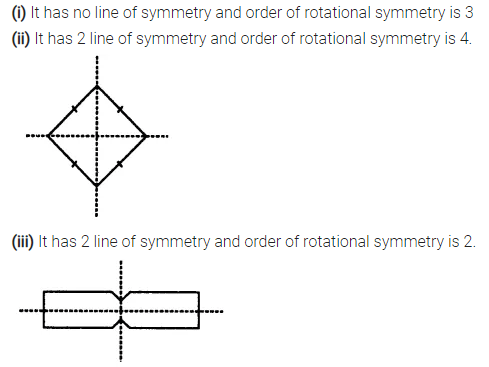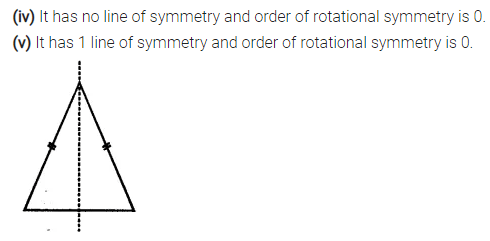# Selina Concise Mathematics Class 7 ICSE Solutions Chapter 17 Symmetry

## Selina Concise Mathematics Class 7 ICSE Solutions Chapter 17 Symmetry (Including Reflection and Rotation)

Selina Publishers Concise Mathematics Class 7 ICSE Solutions Chapter 17 Symmetry (Including Reflection and Rotation)

### Symmetry Exercise 17A – Selina Concise Mathematics Class 7 ICSE Solutions

Question 1.
For each figure, given below, draw the line (s) of symmetry, if possible :Solution:Question 2.
Write capital letters A to Z of English alphabet ; and in each case, if possible, draw the largest number of lines of symmetry.
Solution: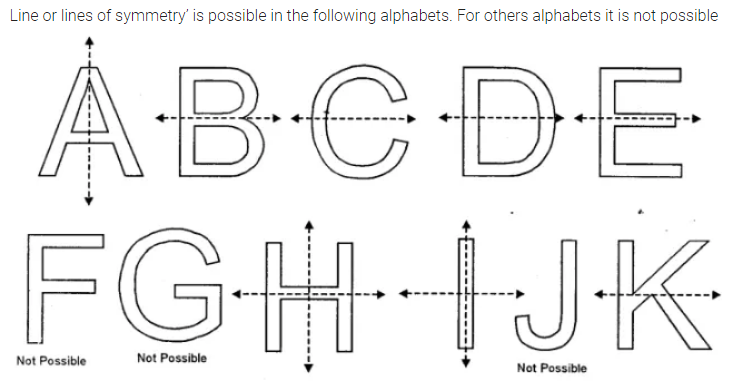Question 3.
By drawing a free hand sketch of each of the following, draw in each case, the line (s) of symmetry,
if any:
(i) a scalene triangle
(ii) an isosceles right angled triangle
(iii) a rhombus
(iv) a kite shaped figure triangle.
(v) a rectangle
(vi) a square
(vii) an isosceles
Solution: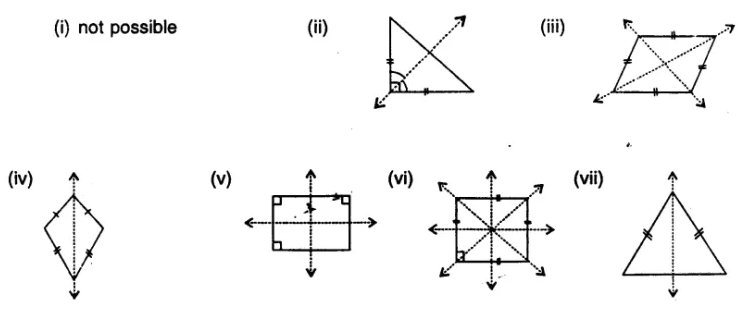Question 4.
Draw a triangle with :
(i) no line of symmetry,
(ii) only one line of symmetry,
(iii) exactly two lines of symmetry,
(iv) exactly three lines of symmetry,
(v) more than three lines of symmetry.
In each case, if possible, represent the line/ lines of symmetry by dotted lines. Also, write the special name of the triangle drawn.
Solution: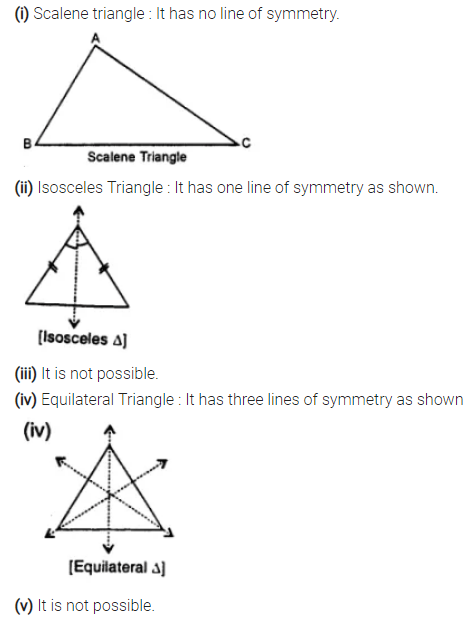Question 5.
(i) no line of symmetry.
(ii) only one line of symmetry.
(iii) exactly two lines of symmetry.
(iv) exactly three lines of symmetry.
(v) exactly four lines of symmetry.
(vi) more than four lines of symmetry.
In each case, if possible, represent the line/ lines of symmetry by dotted lines. Also, write the special name of the quadrilateral drawn.
Solution: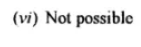Question 6.
Construct an equilateral triangle with each side 6 cm. In the triangle drawn, draw all the possible lines of symmetry.
Solution: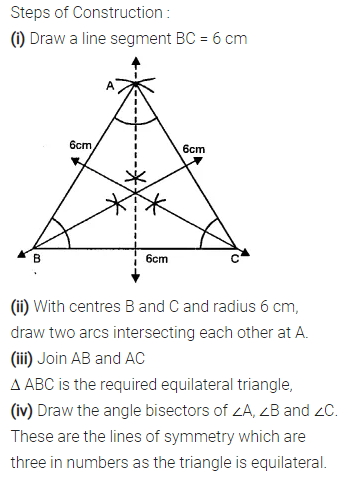Question 7.
Construct a triangle ABC in which AB = AC = 5cin and BC = 5.6 cm. If possible, draw its lines of symmetry.
Solution: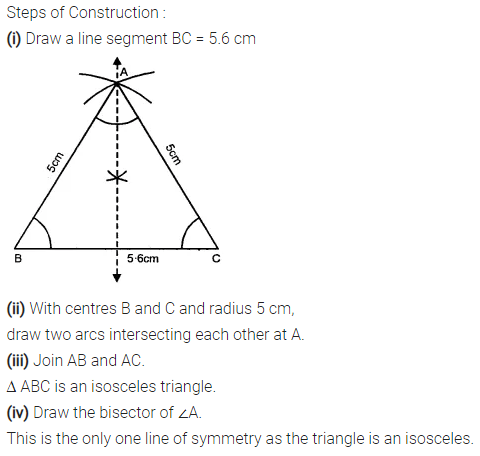Question 8.
Construct a triangle PQR such that PQ = QR = 5 .5 cm and angle PQR = 90°. If possible, draw its lines of symmetry.
Solution: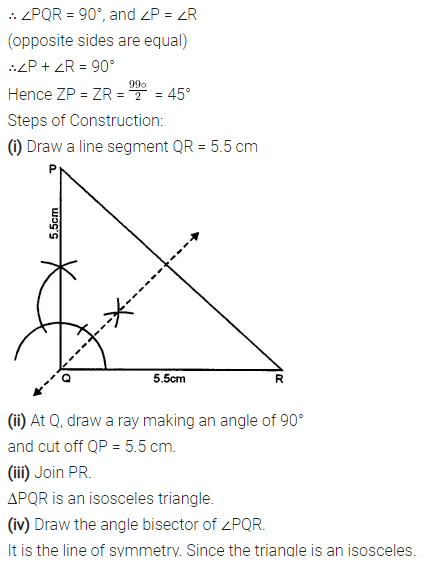Question 9.
If possible, draw a rough sketch of a quadrilateral which has exactly two lines of symmetry.
Solution: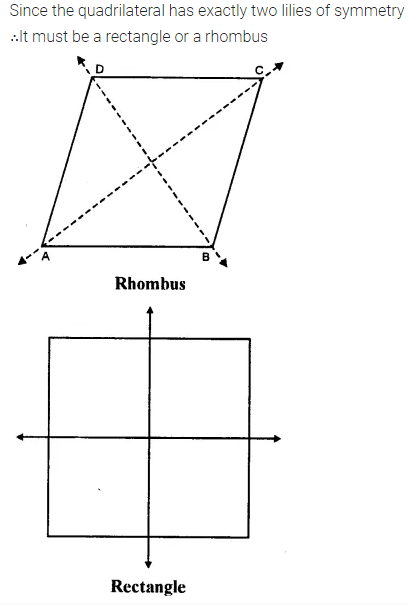Question 10.
A quadrilateral ABCD is symmetric about its diagonal AC. Name tire sides of this quadrilateral which are equal.
Solution:### Symmetry Exercise 17B – Selina Concise Mathematics Class 7 ICSE Solutions

Question 1.
In each figure, given below, find the image of the point P in the line AB :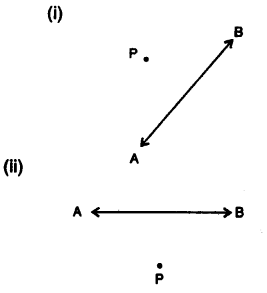Solution: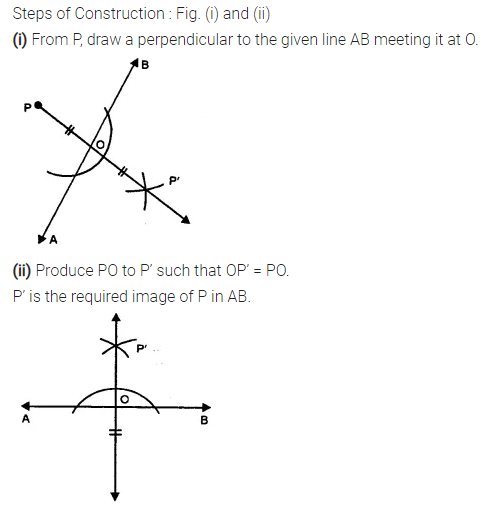Question 2.
In each figure, given below, find the image of the line segment AB in the line PQ :Solution: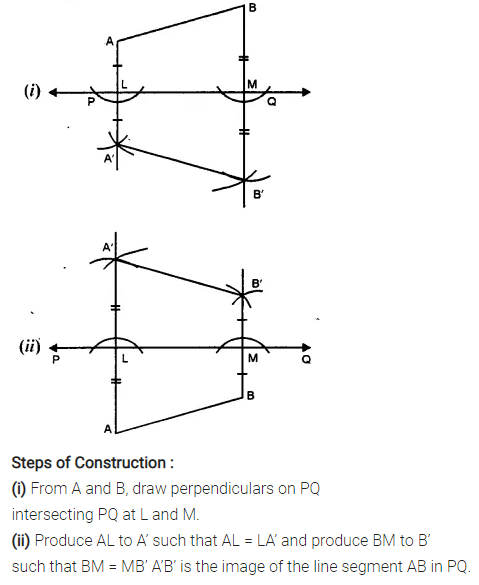Question 3.
Complete the following table :Solution:Question 4.
A point P (7,3)|is reflected in x-axis to point P’. The point P’ is further reflected in v-axis to point P” Find :
(i) the co-ordinates of P’
(ii) the co-ordinates of P”
(iii) the image of P (7, 3) in origin.
Solution:Question 5.
A point A (- 5, 4) is reflected in y-axis to point B. The point B is further reflected in origin to point C. find :
(i) the co-ordinates of B
(ii) the co-ordinates of C
(iii) the image of A (- 5, 4) in x-axis.
Solution:Question 6.
The point P (3, – 8) is reflected in origin to point Q. The Point Q is further reflected in x-axis to point R. Find :
(i) the co-ordinates of Q
(ii) the co-ordinates of R
(iii) the image of P (3, – 8) in y-axis.
Solution:Question 7.
Each of the points A (3, 0), B (7, 0), C (- 8, 0), D (- 7, 0) and E (0, 0) is reflected in x-axis to points A’, B’, C’, D’ and E’ respectively. Write the co-ordinates of each of the image points A’, B’, C’, D’ and E’.
Solution:Question 8.
Each of the points A (0, 4), B (0, 10), C (0, – 4), D (0, – 6) and E (0, 0) is reflected in y-axis to points A’, B’, C’, D’ and E’ respectively. Write the co-ordinates of each of the image points A’, B’, C’, D’ and E’.
Solution:Question 9.
Each of the points A (0, 7), B (8. 0), C (0, -5), D (- 7, 0) and E (0, 0) are reflected in origin to points A’, B’, C’, D’ and E’ respectively. Write the co-ordinates of each of the image points A’, B’, C’, D’ and E’.
Solution: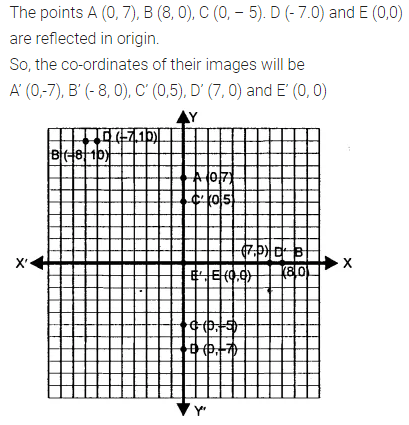Question 10.
Mark points A (4, 5) and B (- 5, 4) on a graph paper. Find A’, the image of A in x-axis and B’, the image of B in x-axis.
Mark A’ and B’ also on the same graph paper.
(ii) Join AB and A’ B’ and
find if AB = A’ B’ ?
Solution: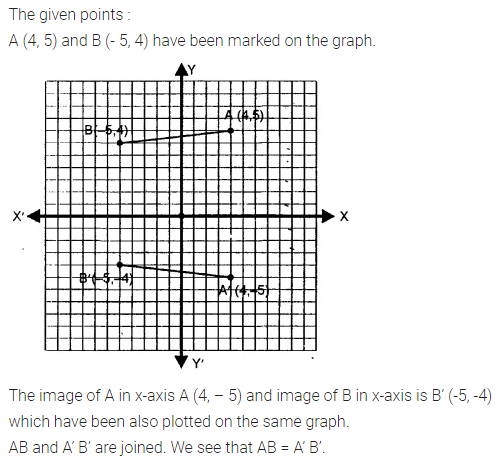Question 11.
Mark points A (6, 4) and B (4, – 6) on a graph paper.
Find A’, the image of A in y-axis and B’, the image of B in y-axis. Mark A’ and B’ also on the same graph paper.
Solution:Question 12.
Mark points A (- 6, 5) and B (- 4, – 6) on a graph paper. Find A’, the image of A in origin and B’, the image of B in origin. Mark A’ and B’ also on the same graph paper. Join AB and A’ B’. Is AB = A’ B’ ?
Solution: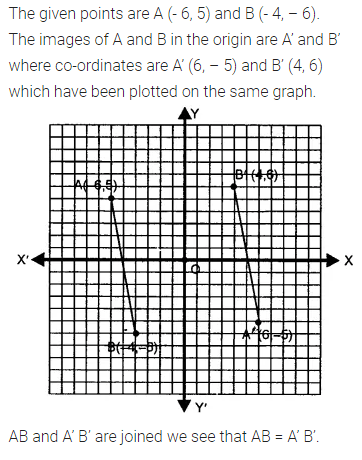### Symmetry Exercise 17C – Selina Concise Mathematics Class 7 ICSE Solutions

Question 1.
How many lines of symmetry does a rhombus have?
Solution: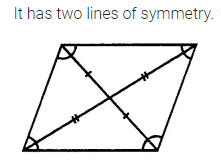Question 2.
What is the order of rotational symmetry of a rhombus?
Solution:Question 3.
Show that each of the following figures has two lines of symmetry and a rotational.Solution:Question 4.
Name a figure that has a line of symmetry but does not have any roational symmetry.
Solution: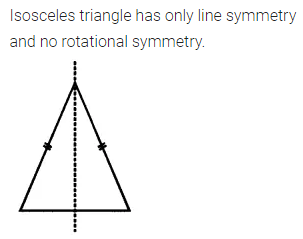Question 5.
In each of the following figures, draw all possible lines of symmetry and also write the order of rotational symmetry:Solution: